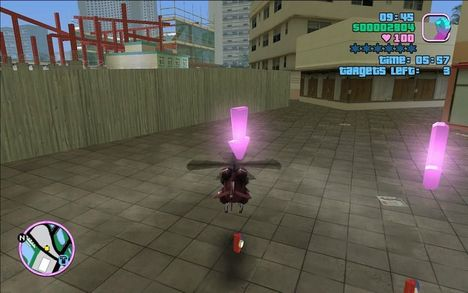# Helikopter HelikopterlonglongintIntra BUET Junior Program...
Limits 1.5s, 512 MBTommy Vercetti has completed the infamous RC helicopter mission after many attempts. And now he needs your help flying a real helicopter.

There are $M$ bombs and $N$ helipads. And there is one Helikopter. Initially all bombs, the Helikopter and Tommy Vercetti are at helipad number $1$. All the bombs need to be transported to helipad $N$. Each bomb $i$ from $1$ to $M$ has a weight of $W_i$.

There is a customs officer on each helipad. Officer $i$ will check the cargo of a Helikopter if and only if the cargo weight exceeds $C_i$ while landing on helipad $i$. Tommy does not want to be caught carrying bombs. And that is why his friend Lance Vance ওরফে কালা জাহাঙ্গীর has built hiding compartments in each helipad to store bombs temporarily.

The following rules are applicable for flying the Helikopter:

1. Taking off from helipad $i$, the Helikopter must land at helipad $i+1$ or $i-1$, which takes $1$ unit of time.

2. Helikopter can carry any number of bombs.

3. While parked on a helipad, Helikopter can drop or pickup any number of bombs from the hiding compartment instantaneously.

If it is possible to transport all bombs to helipad $N$ (without Tommy getting caught of course) then print the minimum time in which it can be done. Otherwise print -1.

## Input

Input is given from Standard Input in the following format:

$N$

$C_1 C_2 … C_N$

$M$

$W_1 W_2 … W_M$

Constraints

• $2 \leq N \leq 5 \times 10^5$

• $1 \leq M \leq 15$

• $1 \leq W_i, C_i \leq 10^{15}$

## Samples

InputOutput
2
10 1
1
2

-1


Tommy will be caught by the officer of helipad $N$.

InputOutput
5
20 20 20 20 20
3
5 5 5

4


Helikopter takes all bombs together in one go.

InputOutput
3
1 1 1
3
1 1 1

10


Helikopter takes bomb $1$ from $1$ to $N$ and then comes back to $1$$\rightarrow$ $4$ moves. (During this, bomb number $2$ and $3$ are in hiding compartment of helipad $1$)

Helikopter takes bomb $2$ from $1$ to $N$ and then comes back to $1$$\rightarrow$ $4$ moves. (During this, bomb number $3$ is in hiding compartment of helipad $1$)

Helikopter takes bomb $3$ from $1$ to $N$ $\rightarrow$ $2$ moves.

Total $10$ moves.

Helikopter, helikopter
Para kofer, para kofer

Helikopter, helikopter
Šaljem ako treba
Para kofer, para kofer
Da bacaš sa neba
Helikopter, helikopter
Kol’ko treba zlata
Samo da od doma otvoriš mi vrata?

Majko moja, kakva je
Stvorena je za mene
Majko moja, vidi je
Vrh ljepote, takva je

Ne postoji blago to
A ni cijena ta
Na koju ne pristajem
Da je imam ja

Helikopter, helikopter
Šaljem ako treba
Para kofer, para kofer
Da bacaš sa nеba
Helikopter, helikoptеr
Kol’ko treba zlata
Samo da od doma otvoriš mi vrata?

Kao da je dragi Bog
Baš za sebe stvorio
Od nje nije vidjelo

Ne postoji blago to
A ni cijena ta
Na koju ne pristajem
Da je imam ja

Helikopter, helikopter
Šaljem ako treba
Para kofer, para kofer
Da bacaš sa neba
Helikopter, helikopter
Kol’ko treba zlata
Samo da od doma otvoriš mi vrata?

### Submit